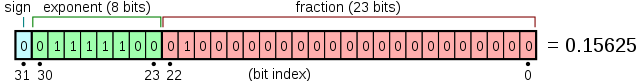August 7, 2022

# Generating Random Floats in [0, 1)

Recently, I found myself in a situation where I needed to generate a random float between 0 and 1 using a random 32-bit integer in C. This subject has been done to death before, but I felt like feeling a little pain so I decided to figure it out myself before using someone else's better solution.

First things first: we actually need to obtain some random integers. Easy, right? We'll just call rand() and—This is what will happen to you if you even suggest using C's rand().

rand() is known to have a number of problems; here's a short list:

• If you use glibc like me, your rand() uses a Linear Congruential Generator. LCGs are very simple, but they are prone to subtle correlation issues that tend to manifest when applied to a multidimensional problem, such as Monte Carlo simulations.
• srand() takes an unsigned int, restricting the number of possible sequences you can get from rand() to number of distinct seeds that you can provide. For reference most quality PRNGs have an internal state of at least 128 bits, which is almost certainly larger than the size of an unsigned int on your platform.
• rand() is not thread safe; calling it will result in reads and writes to a shared location. Not only will this affect performance, it will also cause insidious randomness issues that may choose to emerge at the most inconvenient time. rand_r() allows the user to provide a pointer that will be used to store the PRNG's state, allowing thread-safe code to be written, but many programmers are seemingly not aware of this fact.

Okay, so rand() is not as horrible as I've made it out to be. Don't get me wrong—rand() is pretty crappy, but if you generated 100 random numbers and stared at it, it would probably look pretty random. So rand() might be okay for use cases where high quality randomness is not required (such as a game), but if you try subjecting it to rigorous statistical tests it crumples like wet toilet paper.

Today, we're going to be using xoshiro128+ as our PRNG. Admittedly, the choice of PRNG is not actually that important, which may come as a surprise after my rant about rand(). However, as long as you are not using a crappy or obscure generator, there are plenty of very fast, well-proven generators that are probably sufficient for your application.

# Introducing Floating Point §

Okay, great. So now we have a random uint32_t... how do we turn this into a random float?

The naïve solution is to just divide the integer by the maximum value (232), but this is not great for two reasons:

• Division is slow.
• This requires casting the integer to a float, but floats themselves are also 32 bits, so a float could not possibly represent all 32-bit integers. You can avoid this precision loss by casting to a double, but that just makes the first issue worse.

Instead, what we want to do is create a floating point number using bitwise operations. To do that, we need to familiarize ourselves with how floating point values are represented in memory; specifically, we're going to be focusing on IEEE 754, which is sort of required in the C standard.The anatomy of a single-precision float. Image by Fresheneesz

If you ever learned scientific notation in school, a floating point number is a lot like that. Basically, three fields are packed together: the sign bit, the exponent, and the integer. The function of the sign bit is very simple; 0 is positive and 1 is negative. The real value represented by a float is basically equal to $\mathrm{fraction} \times 2^\mathrm{exponent}$.

There are a few pitfalls that we need to be aware of, however:

• The exponent is stored with an offset of 127, so an exponent of 0 is equal to -127, an exponent of 127 is equal to 0, and an exponent of 255 is equal to 128.
• The fraction section is interpreted as if there was an additional 1 bit in front of it.

In this case, the exponent portion is equal to $124 - 127 = -3$, and the fraction section is equal to $1.01_2 = 1.25$. Thus, the overall value is equal to $2^{-3} \times 1.25 = 0.15625$.

# Actually Generating Floats §

As usual, I've managed to stretch what ought to be a simple blogpost into a long-winded rag about unrelated stuff... bummer. Okay, let's actually generate some floats.

We can start by drawing 23 bits from our PRNG for the fraction part. This is easy enough; we just take a 32-bit value and shift it right 9 bits. This gives us a random fraction value between $1.000\ldots_2 = 1$ and $1.111\ldots_2 \approx 2$.

If we set the exponent portion to 0, the fraction portion will be multipled with $2^0 = 1$, giving us a random number in the range $[1, 2)$. We can easily turn into our desired value in range $[0, 1)$ by subtracting 1.

Here's the actual code:

float int_to_float(uint32_t random) {
union { uint32_t u32; float f; } u = { .u32 = random >> 9 | 0x3f800000 };
return u.f - 1.0;
}

0x3f800000 is what we get when we take our exponent (0 is represented as 127) and shift it right 23 bits so that it's in the right place.

We could use plain old floating point multiplication instead of type punning, though there is a chance that it will be imperceptibly slower than our hacky solution. Probably not. Whatever.

You can find the full code demonstrating this concept here. That's all, folks.